# Fractions + volume - math problems

#### Number of problems found: 49

• Equilateral coneWe pour so much water into a container that has the shape of an equilateral cone, the base of which has a radius r = 6 cm, that one-third of the volume of the cone is filled. How high will the water reach if we turn the cone upside down?
• A bucketA bucket has 4 liters of water in it when it is 2/5 full. How much can it hold?
• Triangular prismThe base of the perpendicular triangular prism is a rectangular triangle with a hypotenuse of 10 cm and one leg of 8 cm. The prism height is 75% of the perimeter of the base. Calculate the volume and surface of the prism.
• Milk2cheese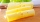From 40 liters of milk is produced 8 kg of cheese how many liters of milk are needed to produce 2 kg of cheese?
• Aircraft angines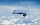The two engines of the aircraft are enough to supply the fuel for five hours of operation. However, one of the engines has a malfunction and thus consumes one-third more fuel. How long can the plane be in the air before it runs out of fuel? After an hour
• Water containerThe cube-shaped container is filled to two-thirds of its height. If we pour 18 liters, it will be filled to three-fifths of the height. What is the volume of the whole container?
• Nádoba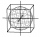Nádoba tvaru kostky je naplněna vodou do poloviny své výšky. Pokud dolijeme 20 l vody, bude nádoba naplněna do tří čtvrtin své výšky. Jaký je objem celé nádoby?
• Tributaries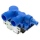The pool can be filled with two different tributaries. The first inflow would fill the pool in 18 hours, both in 6 hours. How many hours would the pool filled with a second inflow?
• Alcohol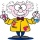6l 48% alcohol, how much 52% alcohol must be added to give 50% alcohol?
• Barrel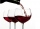Barrel of wine is filled by 9/14. What part of the barrel will remain filled when 1/4 of the wine we pour from the barrel?
• Three glasses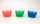Three glasses of different colors have different volumes. Red 1.5 liter is filled from 2/5, blue 3/4 liter is filled from 1/3, and the third green 1.2 liter is empty. Pour green glass 1/4 of the contents from the red glass and 2/5 of the content from the
• Tank of fuelA 14.5-gallon tank of fuel is 3/4 full. How many more gallons will it take to fill up the tank?
• Gasoline-oil ratioThe manufacturer of a scooter engine recommends a gasoline-oil fuel mixture ratio of 15 to 1. In a particular garage, we can buy pure gasoline and a gasoline-oil mixture, which is 75% gasoline. How much gasoline and how much of the gasoline-oil mix do we
• Ratio of volumesIf the heights of two cylindrical drums are in the ratio 7:8 and their base radii are in the ratio 4:3. What is the ratio of their volumes?The radius of the sphere we reduce by 1/3 of the original radius. How much percent does the volume and surface of the sphere change?
• Inlet minus outlet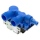Water flows into the tank through two pipes and flows out of the third pipe spontaneously. The pipe A itself would fill the tank in 3 hours, the pipe B itself would fille in 4 hours, and the pipe C itself would flow out in 12 hours. How long will the tank
• Cube cutIn the ABCDA'B'C'D'cube, it is guided by the edge of the CC' a plane witch dividing the cube into two perpendicular four-sided and triangular prisms, whose volumes are 3:2. Determine in which ratio the edge AB is divided by this plane.
• HectolitersHow deep is the pool if there are 2025 hectoliters of water and the bottom dimensions are a = 15 meters b = 7,5 meters and the water level is up to 9/10 (nine-tenths) of height.
• BucketThe bucket half filled with water weighs 5.55 kg, the full bucket weighs 9.85 kg. How much does the bucket weigh?
• Soup from canteen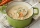For how many people is 90 liters of soup enough if we assume 3/8 liter of soup per person in the canteen?

Do you have an interesting mathematical word problem that you can't solve it? Submit a math problem, and we can try to solve it.

We will send a solution to your e-mail address. Solved examples are also published here. Please enter the e-mail correctly and check whether you don't have a full mailbox.

Please do not submit problems from current active competitions such as Mathematical Olympiad, correspondence seminars etc...

Need help calculate sum, simplify or multiply fractions? Try our fraction calculator. Tip: Our volume units converter will help you with the conversion of volume units.